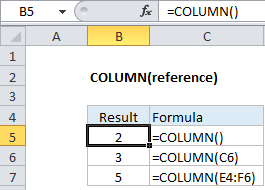## Excel Office

Excel How Tos, Tutorials, Tips & Tricks, Shortcuts

# How to use Excel COLUMN Function

This Excel tutorial explains how to use the COLUMN function with syntax and examples.

## Excel COLUMN function Description

The Microsoft Excel COLUMN function returns the column number of a cell reference.

The COLUMN function is a built-in function in Excel that is categorized as a Lookup/Reference Function. It can be used as a worksheet function in Excel. As a worksheet function, the COLUMN function can be entered as part of a formula in a cell of a worksheet.

Worked Example:   How to get last column number in range in ExcelExplanation: The Excel COLUMN function returns the column number for a reference. For example, COLUMN(C5) returns 3, since C is the third column in the spreadsheet. When no reference is provided, COLUMN returns the column number of the cell which contains the formula.

Worked Example:   How to get address of named range in Excel

### Syntax

The syntax for the COLUMN function in Microsoft Excel is:

`COLUMN( [reference] )`

### Returns

The COLUMN function returns a numeric value.

Worked Example:   Sum every nth column in Excel